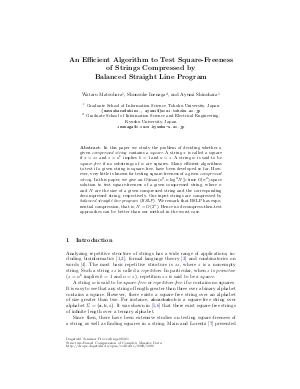Document# An Efficient Algorithm to Test Square-Freeness of Strings Compressed by Balanced Straight Line Program

### Authors Wataru Matsubara, Shunsuke Inenaga, Ayumi Shinohara## File

DagSemProc.08261.5.pdf
• Filesize: 344 kB
• 19 pages

## Cite As

Wataru Matsubara, Shunsuke Inenaga, and Ayumi Shinohara. An Efficient Algorithm to Test Square-Freeness of Strings Compressed by Balanced Straight Line Program. In Structure-Based Compression of Complex Massive Data. Dagstuhl Seminar Proceedings, Volume 8261, Schloss Dagstuhl - Leibniz-Zentrum für Informatik (2008)
https://doi.org/10.4230/DagSemProc.08261.5

## Abstract

In this paper we study the problem of deciding whether a given compressed string contains a square. A string x is called a square if x = zz and z = u^k implies k = 1 and u = z. A string w is said to be square-free if no substrings of w are squares. Many efficient algorithms to test if a given string is square-free, have been developed so far. However, very little is known for testing square-freeness of a given compressed string. In this paper, we give an O(max(n^2; n log^2 N))-time O(n^2)-space solution to test square-freeness of a given compressed string, where n and N are the size of a given compressed string and the corresponding decompressed string, respectively. Our input strings are compressed by balanced straight line program (BSLP). We remark that BSLP has exponential compression, that is, N = O(2^n). Hence no decompress-then-test approaches can be better than our method in the worst case.
##### Keywords
• Square Freeness
• Straight Line Program

## Metrics

• Access Statistics
• Total Accesses (updated on a weekly basis)
0